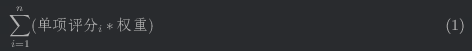spacemacs org中得latex fragment里面输入中文preview并不正确

#1
\begin{equation}
\sum\limits_{i=1}^n(单项评分_i * 权重)
\end{equation}

#2

\begin{equation}
\sum\limits_{i=1}^n(\text{单项评分}_i * \text{权重})
\end{equation}#3#4

latex 的 math mode 本来就是不能显示 CJK 字体的， 需要引入 xeCJK 这些处理中文的包， 可能你需要添加 #+latex_header

#5

(setq org-latex-default-packages-alist '(("T1" "fontenc" t ("pdflatex"))
("" "graphicx" t)
("" "grffile" t)
("" "longtable" nil)
("" "wrapfig" nil)
("" "rotating" nil)
("normalem" "ulem" t)
("" "amsmath" t)
("" "textcomp" t)
("" "amssymb" t)
("" "capt-of" nil)
("" "hyperref" nil)))
(setq org-latex-packages-alist '(("fontset=windowsnew,UTF8" "ctex" t)
("range-phrase=-,range-units=single" "siunitx" t)
("" "upgreek" t)))

;; preview latex settings
(setq org-preview-latex-default-process 'imagemagick)

#6

#7

#8

(setq org-latex-packages-alist
'(("fontset=macnew,UTF8" "ctex" t)))

(setq org-preview-latex-default-process 'dvisvgm)
(setq org-preview-latex-process-alist
'((dvisvgm :programs
("xelatex" "dvisvgm")
:description "xdv > svg" :message "you need to install the programs: xelatex and dvisvgm." :use-xcolor t :image-input-type "xdv" :image-output-type "svg" :image-size-adjust
(1.7 . 1.5)
:latex-compiler
("xelatex -no-pdf -interaction nonstopmode -output-directory %o %f")
:image-converter
("dvisvgm %f -n -b min -c %S -o %O"))
(imagemagick :programs
("xelatex" "convert")
:description "pdf > png" :message "you need to install the programs: xelatex and imagemagick." :use-xcolor t :image-input-type "pdf" :image-output-type "png" :image-size-adjust
(1.0 . 1.0)
:latex-compiler
("xelatex -interaction nonstopmode -output-directory %o %f")
:image-converter
("convert -density %D -trim -antialias %f -quality 100 %O"))))

#9

DVI error: DVI format 6 not supported File mode specification error: (error File “/tmp/orgtex3AETPu.svg” wasn’t produced. Please adjust ‘dvisvgm’ part of ‘org-preview-latex-process-alist’.)

#10

#11

#12

#13

\begin{equation}
\sum\limits_{i=1}^n(\text{单项评分}_i * \text{权重})
\end{equation}